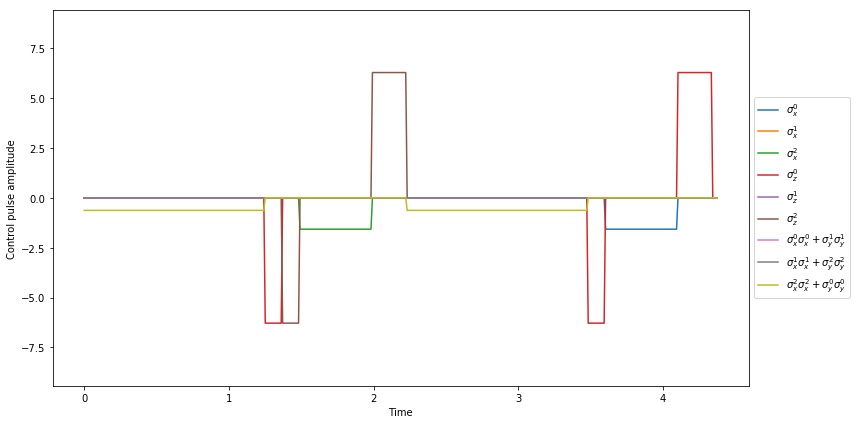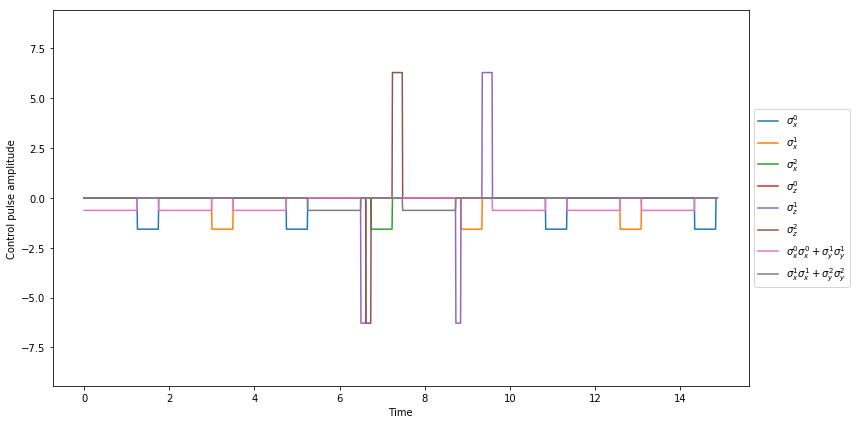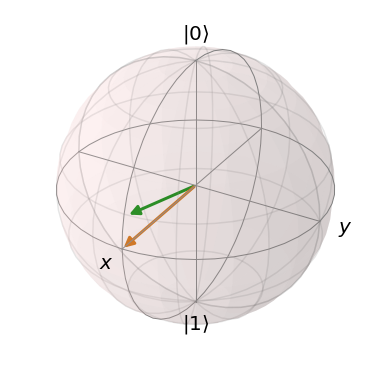# QuTiP example: Physical implementation of Spin Chain Qubit model¶

Author: Anubhav Vardhan ([email protected])

Numerical simulation added by Boxi Li ([email protected])

For more information about QuTiP see http://qutip.org

In :
%matplotlib inline

In :
from qutip.qip.circuit import QubitCircuit
from qutip.qip.operations import gate_sequence_product
import numpy as np


If your qutip version is lower than 4.4.1 please run the following cell

In [ ]:
from qutip.qip.models.spinchain import CircularSpinChain
from qutip.qip.models.spinchain import LinearSpinChain


Otherwise please run this cell

In :
from qutip.qip.device import CircularSpinChain, LinearSpinChain
from qutip.qip.noise import RandomNoise


## Hamiltonian:¶

$\displaystyle H = - \frac{1}{2}\sum_n^N h_n \sigma_z(n) - \frac{1}{2} \sum_n^{N-1} [ J_x^{(n)} \sigma_x(n) \sigma_x(n+1) + J_y^{(n)} \sigma_y(n) \sigma_y(n+1) +J_z^{(n)} \sigma_z(n) \sigma_z(n+1)]$

The linear and circular spin chain models employing the nearest neighbor interaction can be implemented using the SpinChain class.

## Circuit Setup¶

In :
N = 3
qc = QubitCircuit(N)

qc.add_gate("CNOT", targets=, controls=)


The non-adjacent interactions are broken into a series of adjacent ones by the program automatically.

In :
U_ideal = gate_sequence_product(qc.propagators())

U_ideal

Out:
Quantum object: dims = [[2, 2, 2], [2, 2, 2]], shape = (8, 8), type = oper, isherm = True\begin{equation*}\left(\begin{array}{*{11}c}1.0 & 0.0 & 0.0 & 0.0 & 0.0 & 0.0 & 0.0 & 0.0\\0.0 & 0.0 & 0.0 & 0.0 & 0.0 & 1.0 & 0.0 & 0.0\\0.0 & 0.0 & 1.0 & 0.0 & 0.0 & 0.0 & 0.0 & 0.0\\0.0 & 0.0 & 0.0 & 0.0 & 0.0 & 0.0 & 0.0 & 1.0\\0.0 & 0.0 & 0.0 & 0.0 & 1.0 & 0.0 & 0.0 & 0.0\\0.0 & 1.0 & 0.0 & 0.0 & 0.0 & 0.0 & 0.0 & 0.0\\0.0 & 0.0 & 0.0 & 0.0 & 0.0 & 0.0 & 1.0 & 0.0\\0.0 & 0.0 & 0.0 & 1.0 & 0.0 & 0.0 & 0.0 & 0.0\\\end{array}\right)\end{equation*}

## Circular Spin Chain Model Implementation¶

In :
p1 = CircularSpinChain(N, correct_global_phase=True)

U_list = p1.run(qc)

U_physical = gate_sequence_product(U_list)

U_physical.tidyup(atol=1e-5)

Out:
Quantum object: dims = [[2, 2, 2], [2, 2, 2]], shape = (8, 8), type = oper, isherm = True\begin{equation*}\left(\begin{array}{*{11}c}1.0 & 0.0 & 0.0 & 0.0 & 0.0 & 0.0 & 0.0 & 0.0\\0.0 & 0.0 & 0.0 & 0.0 & 0.0 & 1.0 & 0.0 & 0.0\\0.0 & 0.0 & 1.0 & 0.0 & 0.0 & 0.0 & 0.0 & 0.0\\0.0 & 0.0 & 0.0 & 0.0 & 0.0 & 0.0 & 0.0 & 1.0\\0.0 & 0.0 & 0.0 & 0.0 & 1.0 & 0.0 & 0.0 & 0.0\\0.0 & 1.0 & 0.0 & 0.0 & 0.0 & 0.0 & 0.0 & 0.0\\0.0 & 0.0 & 0.0 & 0.0 & 0.0 & 0.0 & 1.0 & 0.0\\0.0 & 0.0 & 0.0 & 1.0 & 0.0 & 0.0 & 0.0 & 0.0\\\end{array}\right)\end{equation*}
In :
(U_ideal - U_physical).norm()

Out:
0.0

The results obtained from the physical implementation agree with the ideal result.

In :
p1.qc0.gates

Out:
[Gate(CNOT, targets=, controls=)]

The gates are first convert to gates with adjacent interactions moving in the direction with the least number of qubits in between.

In :
p1.qc1.gates

Out:
[Gate(CNOT, targets=, controls=)]

They are then converted into the basis [ISWAP, RX, RZ]

In :
p1.qc2.gates

Out:
[Gate(GLOBALPHASE, targets=None, controls=None),
Gate(ISWAP, targets=[2, 0], controls=None),
Gate(RZ, targets=, controls=None),
Gate(RZ, targets=, controls=None),
Gate(RX, targets=, controls=None),
Gate(RZ, targets=, controls=None),
Gate(RZ, targets=, controls=None),
Gate(ISWAP, targets=[2, 0], controls=None),
Gate(RZ, targets=, controls=None),
Gate(RX, targets=, controls=None),
Gate(RZ, targets=, controls=None),
Gate(RZ, targets=, controls=None)]

The time for each applied gate:

In :
p1.get_full_tlist()

Out:
array([0.   , 1.25 , 1.375, 1.5  , 2.   , 2.125, 2.25 , 3.5  , 3.625,
4.125, 4.25 , 4.375])

The pulse can be plotted as:

In :
p1.plot_pulses();## Linear Spin Chain Model Implementation¶

In :
p2 = LinearSpinChain(N, correct_global_phase=True)

U_list = p2.run(qc)

U_physical = gate_sequence_product(U_list)

U_physical.tidyup(atol=1e-5)

Out:
Quantum object: dims = [[2, 2, 2], [2, 2, 2]], shape = (8, 8), type = oper, isherm = True\begin{equation*}\left(\begin{array}{*{11}c}1.000 & 0.0 & 0.0 & 0.0 & 0.0 & 0.0 & 0.0 & 0.0\\0.0 & 0.0 & 0.0 & 0.0 & 0.0 & 1.000 & 0.0 & 0.0\\0.0 & 0.0 & 1.000 & 0.0 & 0.0 & 0.0 & 0.0 & 0.0\\0.0 & 0.0 & 0.0 & 0.0 & 0.0 & 0.0 & 0.0 & 1.0\\0.0 & 0.0 & 0.0 & 0.0 & 1.0 & 0.0 & 0.0 & 0.0\\0.0 & 1.0 & 0.0 & 0.0 & 0.0 & 0.0 & 0.0 & 0.0\\0.0 & 0.0 & 0.0 & 0.0 & 0.0 & 0.0 & 1.0 & 0.0\\0.0 & 0.0 & 0.0 & 1.0 & 0.0 & 0.0 & 0.0 & 0.0\\\end{array}\right)\end{equation*}
In :
(U_ideal - U_physical).norm()

Out:
0.0

The results obtained from the physical implementation agree with the ideal result.

In :
p2.qc0.gates

Out:
[Gate(CNOT, targets=, controls=)]

The gates are first convert to gates with adjacent interactions moving in the direction with the least number of qubits in between.

In :
p2.qc1.gates

Out:
[Gate(SWAP, targets=[0, 1], controls=None),
Gate(CNOT, targets=, controls=),
Gate(SWAP, targets=[0, 1], controls=None)]

They are then converted into the basis [ISWAP, RX, RZ]

In :
p2.qc2.gates

Out:
[Gate(GLOBALPHASE, targets=None, controls=None),
Gate(ISWAP, targets=[0, 1], controls=None),
Gate(RX, targets=, controls=None),
Gate(ISWAP, targets=[0, 1], controls=None),
Gate(RX, targets=, controls=None),
Gate(ISWAP, targets=[1, 0], controls=None),
Gate(RX, targets=, controls=None),
Gate(GLOBALPHASE, targets=None, controls=None),
Gate(ISWAP, targets=[2, 1], controls=None),
Gate(RZ, targets=, controls=None),
Gate(RZ, targets=, controls=None),
Gate(RX, targets=, controls=None),
Gate(RZ, targets=, controls=None),
Gate(RZ, targets=, controls=None),
Gate(ISWAP, targets=[2, 1], controls=None),
Gate(RZ, targets=, controls=None),
Gate(RX, targets=, controls=None),
Gate(RZ, targets=, controls=None),
Gate(RZ, targets=, controls=None),
Gate(GLOBALPHASE, targets=None, controls=None),
Gate(ISWAP, targets=[0, 1], controls=None),
Gate(RX, targets=, controls=None),
Gate(ISWAP, targets=[0, 1], controls=None),
Gate(RX, targets=, controls=None),
Gate(ISWAP, targets=[1, 0], controls=None),
Gate(RX, targets=, controls=None)]

The time for each applied gate:

In :
p2.get_full_tlist()

Out:
array([ 0.   ,  1.25 ,  1.75 ,  3.   ,  3.5  ,  4.75 ,  5.25 ,  6.5  ,
6.625,  6.75 ,  7.25 ,  7.375,  7.5  ,  8.75 ,  8.875,  9.375,
9.5  ,  9.625, 10.875, 11.375, 12.625, 13.125, 14.375, 14.875])

The pulse can be plotted as:

In :
p2.plot_pulses();## Numerical simulation¶

From QuTiP 4.5, we also add the possibility to allow numerical simulation of SpinChain-based quantum computing. One needs only to add an option analytical=False in run_state to use one of the QuTiP solvers to simulate the state evolution instead of direct matrix product. Under numerical simulation, one can go beyond simulation with perfect gate operations. All the noise defined for the class Processor can also be used for SpinChain here.

In :
from qutip import basis, fidelity
N = 1
plus_state = (basis(2,0) + basis(2,1)).unit()

qc = QubitCircuit(N=N)
qc.add_gate("SNOT", targets=0)
processor = LinearSpinChain(N=N)
processor.load_circuit(qc)
end_state = processor.run_state(init_state=basis(2, 0), analytical=False).states[-1]
fidelity(end_state, plus_state)

Out:
1.0000000052671834
In :
processor.add_noise(RandomNoise(rand_gen=np.random.normal, dt=0.1, loc=0.1, scale=0.2))
end_state = processor.run_state(init_state=basis(2, 0), analytical=False).states[-1]
fidelity(end_state, plus_state)

Out:
0.9915047030474844

As the control noise is coherent noise, the result of this noise is still a pure state. Therefore, we can visualize it on a Bloch sphere.

In :
from qutip.bloch import Bloch
b = Bloch()
b.add_states([end_state, plus_state])
b.make_sphere()### Software versions:¶

In :
from qutip.ipynbtools import version_table
version_table()

Out:
SoftwareVersion
QuTiP4.5.0.dev0+c50c4bc9
Numpy1.16.2
SciPy1.2.1
matplotlib3.0.3
Cython0.29.12
Number of CPUs12
BLAS InfoINTEL MKL
IPython7.4.0
Python3.7.3 (default, Mar 27 2019, 17:13:21) [MSC v.1915 64 bit (AMD64)]
OSnt [win32]
Sun Aug 04 16:45:29 2019 W. Europe Daylight Time
In [ ]: